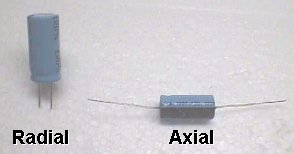# What's My Capacitor?

## How To Heck Do I Know The Value? How To Read A Cap

By Bob Roberts

 Here is an another area that newbies & old hands alike run into trouble with :-( I'm always asked how to figure out what a cap in hand has for a value. It has just 2 numbers on it... it has 3 numbers & a letter... what to heck is it? Caps are coded more or less the same as resistors are but using numerals instead of colors. Use the first 2 numbers as-is & the third is the multiplier. Of course, back in the day they were color coded using the same code as resistors, either with domino dots of color, dashes of color or actual complete bands much like the resistors are today. If you're restoring an old jukebox or anything from days gone by, you may run into some of these older caps, so knowing the color code should be helpful. As always, I hate getting into the technical aspects because I know this is your hobby & all you want to do is be able to read the common caps for replacement & could give a hoot about all the techie stuff, so I'll just give you the basics & then list some of the commonly used caps stated in various ways. Capacitors are measured in farads, but 1 farad is much larger than anything used in coin-op games or any other small electronics for that matter, so we start out with fractions of a farad. I'll put the divisions below, all of which can be crossed into another division, & the abbreviations can be either lower case or upper case. They started out as a mix of both, but have become common place either way over time. picofarad 1 trillionth = pf = pF = uuf = mmf = mmfd = 1/1,000 000 000 000 nanofarad 1 billionth = nf = nF = 1/1,000 000 000 microfarad 1 millionth = uf = uF = mf = 1/1,000 000 millifarad 1 thousandth = 1/1000 = Too Big For Us K... how do we figure out the value? Let's start with caps with 2 numbers on them. These are assumed to be picofarads & if you have a letter it is the tolorance, typically a K for 10% +/-. I don't know of any app that we use that we would have to be concerned with this letter. Many are fooled by the K thinking it is like K for kilo with resistors... don't make that mistake or you'll be way off the mark with your value. Examples 22 = 22pf = .022nf = .000022uf 33 = 33pf = .033nf = .000033uf 56 = 56pf = .056nf = .000056uf 68 = 68pf = .068nf = .000068uf Three numbers... first two as is & the third is the mutiplier with the results in picofarads. It seems to be easier for newbies to think of the multipliers as just the number of zeros to add to the first two numbers. Examples 104 = 10 0000pf = 100nf = .1uf 103 = 10 000pf = 10nf = .01uf 105 = 10 00000pf = 1000nf = 1.0uf 503 = 50 000pf = 50nf = .05uf 682 = 68 00pf = 68nf = .0068uf 822 = 82 00pf = 8.2nf = .0082uf Going from picofarads to microfarads is just simply moving the decimal point 6 positions. Examples 50000pf = .050000uf = .05uf 330pf = .000330uf = .00033uf 22pf = .000022uf Think you have a handle on it? Here's a curve ball.... some mfrs put the value on the cap in ufs, so .47K now reads in ufs & not pfs. When the decimal point is on the cap it reads in microfarads. Hopefully, this has armed you enough to determine what value cap you have. April 2007 Addendum Here's a simple Q that is asked so many times per week that I am compelled to place the answer here: How do I tell if I need radial or axial caps? Radial caps are ones where both leads come from one end & axial caps are ones that have a lead on each end.Happy Gaming...